Polar Coordinates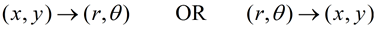Let's start by examining the commands used to convert between rectangular and polar coordinates:
Our primary menu of commands is listed under ANGLE.
Press 2nd APPS (ANGLE) to open this menu.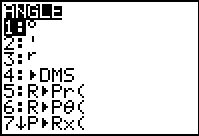Change Rectangular to Polar: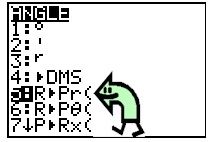Option #5: R►P r is the rectangular to polar conversion
that returns only the r value.
Format:
R►P r (x,y)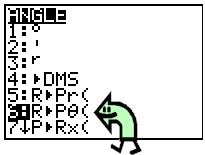Option #6: R►P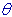( is the rectangular to polar conversion
that returns only thevalue.
Format:
RP(x,y)

 Change Polar  to Rectangular:Option #7:  P►Rx ( is the
polar to rectangular conversion that returns the x value.

Format:   P►Rx (x,y)Option #8:  P►Ry ( is the
polar to rectangular conversion that returns the y value.
Format:
P►Ry (x,y)

Examples:
1.  Convert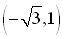to polar form.
We need to find the corresponding (r,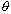) values for the given (x,y) values.

Options #5: R►P r and
#6: R
P(  give: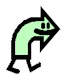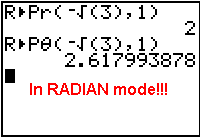NOTE: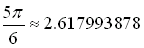Answer:  (2, 2.618) or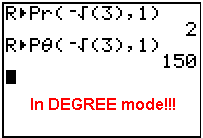________________________________________________________________

2.  Convert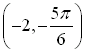to rectangular form.
We need to find the corresponding (x,y) values for the given (r,) values.

Options #7:  P►Rx ( and
#8:  P►Ry (  give: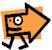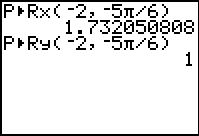NOTE: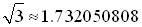Answer:  (1.732, 1)  or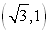Since the stated coordinates are expressed in RADIAN form, set the calculator to RADIAN mode.